# NCERT Solutions for Class 10 Science Magnetic Field Of current Exercise

Question 1.
Which of the following correctly describes the magnetic force near a long straight wire?
(a) The field consists of straight lines perpendicular to the wire.
(b) The field consists of straight lines parallel to the wire.
(c) The field consists of radial lines originating from the wire.
(d) The field consists of concentric circles centred on the wire.
Solution
(d) The magnetic field lines due to a straight current carrying wire are concentric circles, i. e., the field consists of concentric circles centred on the wire.

Question 2.
The phenomenon of electromagnetic induction is
(a) the process of charging a body.
(b) the process of generating magnetic field due to current passing through coil.
(c) the process of producing induced current in coil due to relative motion between a magnet and the coil.
(d) the process of rotating a coil of an electric motor.
Solution
(c) The process of producing induced current in coil due to relative motion between a magnet and the coil.

Question 3.
The device used for producing electric current is called
(a) generator
(b) galvanometer
(c) ammeter
(d) motor
Solution
(a) Generator

Question 4.
The essential difference between AC generator and DC generator is that:
(a) AC generator has electromagnet while DC generator has permanent magnet.
(b) DC generator higher voltage.
(c) AC generates higher voltage
(d) Ac generator has slip rings while DC generator has commutator.
Solution
(d) AC generator has slip rings while DC generator has commutator. Due to slip ring rings, the current produced by AC generator flows in both directions while current produced by DC generator flows sin single direction.

Question 5.
At the time of short circuit, the current in the circuit
(a) reduces substantially.
(b) does not change.
(c) increases heavily.
(d) vary continuously.
Solution
(c)

Question 6.
State whether the following statements are true of false.
(a) An electric motor converts mechanical energy into electrical energy.
(b) An electric generator works on the principle of electromagnetic induction.
(c) Field at the centre of a long circular coil carrying current will be parallel.
(d) Wire with a green insulation is usually the live wire of an electric supply.
Solution
a. False, electric motor converts electric energy into mechanical energy.
b. True
c. True
d. False, the wire with green insulation is the earth wire not the live wire.

Question 7.
List three methods of producing magnetic fields.
Solution
Following are the methods of producing magnetic field:
(a) Passing electric current through a straight conductor/circuit.
(b) Passing electric current through a circular loop.
(c) Passing electric current through a solenoid.

Question 8.
How does a solenoid behave like a magnet? Can you determine the north and south poles of a current carrying solenoid using a bar magnet? Explain.
Solution
A solenoid behaves like a magnet when electric current passes through it. Such a solenoid is called electromagnet. One end of a solenoid behaves as a north pole and the other end behaves as a south pole. We can use a bar magnet to determine the north and south poles of a current carrying solenoid by using property of like poles to repel and unlike poles to attract each other. The end of solenoid which attracts North Pole of a bar magnet is magnetic South Pole in a solenoid. The end which repels the north pole of bar magnet is the magnetic north pole of solenoid.

Question 9.
When the force experienced by a current carrying conductor placed in magnetic is fielded largest?
Solution
The force experienced by a current carrying conductor placed in a magnetic field is the largest when conductor is kept perpendicular to the direction of the magnetic field.
Force acting on current carrying conductor F = Bli sin θ, F will be maximum whey sinθ is maximum. Sin θ max = 1 = sin 900 i. e., conductor is perpendicular to the field.
Question 10
.Imagine that you are sitting in a chamber with your back to one wall. An electron beam, moving horizontally from back wall towards the front wall, is deflected by a strong magnetic field to your right side. What is the direction of magnetic  field?
Solution
The direction of electron are from back wall towards front wall.So current will from front wall to back wall. The direction of the force is towards right According to Fleming’s left hand rule, direction of magnetic field will be vertically downward.

Question 11
Draw a labelled diagram of an electric motor. Explain its principle and working.What is the function of a split ring in an electric motor?
Solution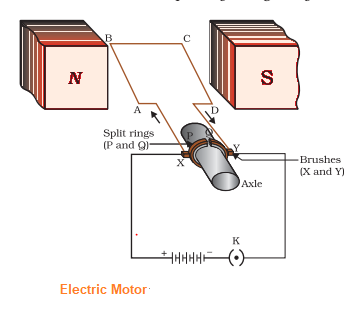Principle of Electric Motor
An electric motor works on the principle that when a current carrying coil is placed in a magnetic field, a force acts on it and tends to rotate the coil.Electric Motor converts electrical energy into mechanical energy
Working of electric Motor
a. An electric motor, consists of a rectangular coil ABCD of insulated copper wire. The coil is placed between the two poles of a magnetic field such that the arm AB and CD are perpendicular to the direction of the magnetic field. The ends of the coil are connected to the two halves P and Q of a split ring.
b. When the electric current is switched on, Current in the coil ABCD enters through conducting brush X and flows back to the battery through brush Y. Current in arm AB of the coil flows from A to B. In arm CD it flows from C to D, that is, opposite to the direction of current through arm AB. On applying Fleming�s left hand rule for the direction of force on a current-carrying conductor in a magnetic field We find that the force acting on arm AB pushes it downwards while the force acting on arm CD pushes it upwards. Thus the coil and the axle O, mounted free to turn about an axis, rotate anti-clockwise.
c.At half rotation, Q makes contact with the brush X and P with brush Y. Therefore the current in the coil gets reversed and flows along the path DCBA. A device that reverses the direction of flow of current through a circuit is called a commutator. In electric motors, the split ring acts as a commutator. The reversal of current also reverses the direction of force acting on the two arms AB and CD. Thus the arm AB of the coil that was earlier pushed down is now pushed up and the arm CD previously pushed up is now pushed down. Therefore the coil and the axle rotate half a turn more in the same direction. The reversing of the current is repeated at each half rotation, giving rise to a continuous rotation of the coil and to the axle.

function of a split ring in an electric motor
split ring acts as a commutator in electric motor. The purpose of the commutator is to reverses the direction of flow of current through a circuit

Question 12.
Name some devices in which electric motors are used.
Solution
Electric motors are used in electric fans, refrigerator, mixtures, washing machines, computers, MP3 players etc.

Question 13.
A coil of insulated copper wire is connected to a galvanometer. What will happen if a bar magnet is (i) pushed into the coil, (ii) withdrawn from inside the coil and (iii) held stationary inside the coil?
Solution
(i) The galvanometer shows a deflection, which means current is induced in the coil. Current is induced in the coil due to the relative motion between coil and magnet.
(ii)The galvanometer shows a deflection in opposite direction, which means current is induced in opposite direction. In this case, the direction of motion is in the opposite direction w r.t. coil.
(iii) There is no deflection in galvanometer as no current is induced in the coil. There is no relative motion between coil and current, so on current will be induced in the coil.

Question 14.
Two circular coils A and B are placed close to each other. If the current in coil A to changed, will some current be induced in the coil? Give reason.
Solution
Yes, current will be induced in coil B. when the current in coil A is changed, the magnetic field around it changes. So, the changing, magnetic filed lines around coil B induce a current in coil B.

Question 15.
State the rule to determine the direction of a
(i) magnetic field produced around a straight conductor carrying current.
(ii) force experienced by a current carrying straight conductor placed in a magnetic field.
(iii) current induced in a coil due to its rotation in magnetic field.
Solution
(i) Ampere’s right hand thumb rule: If current is passing through a conductor in the direction of thumb of the right hand, then the direction of magnetic field is given by that of curvature of fingers.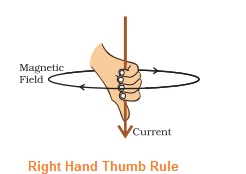(ii)Fleming’s left hand rule: If the forefinger, centre finger and thumb of left hand are starched mutually perpendicular and forefinger points in the direction of magnetic field, centre finger points in the direction of flow of electric current, then thumb points in the direction of force acting on the conductor.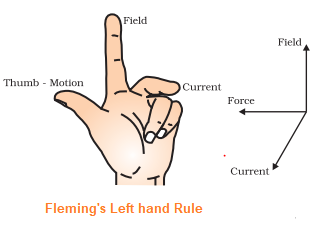(iii)Fleming’s right hand rule: If the forefinger, centre finger and thumb of right hand are stretched mutually perpendicular and forefinger points in direction of magnetic field, thumb points in the direction of motion of conductor, then centre finger points in the direction of induced current.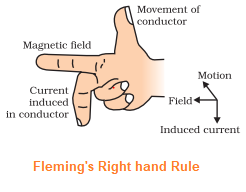Question 16
Explain the underlying principle and working of an electric generator by drawing labelled diagram. What is the function of brushes?
Solution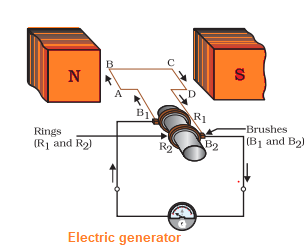Principle of Electric Generator
Electric Generator operates on the principle of electromagnetic induction.When an coil is rotated in magnetic field, an induced current is produced in the circuit and direction of induced current is given by Fleming’s right hand rule. Electric generator converts mechanical energy into electrical energy

Working of electric Generator
a. When the coil ABCD is rotated clockwise . By applying Fleming�s right-hand rule, the induced currents are set up in these arms along the directions AB and CD. Thus an induced current flows in the direction ABCD. This means that the current in the external circuit flows from B2 to B1.
b. After half a rotation, arm CD starts moving up and AB moving down. As a result, the directions of the induced currents in both the arms change, giving rise to the net induced current in the direction DCBA. The current in the external circuit now flows from B1 to B2. Thus after every half rotation the polarity of the current in the respective arms changes. Such a current, which changes direction after equal intervals of time, is called an alternating current (abbreviated as AC). This device is called an AC generator.
c. To get a direct current (DC, which does not change its direction with time), a split-ring type commutator must be used. With this arrangement, one brush is at all times in contact with the arm moving up in the field, while the other is in contact with the arm moving down. Thus a unidirectional current is produced. The generator is thus called a DC generator.

Question 17.
When does an electric short circuit occur?
Solution
When the insulation of wires is damaged or there is a fault in the appliance, due to which the live wire and neutral wire come in direct contact, the current in the circuit increases abruptly. This is called short – circuiting.

Question 18.
What is the function of an earth wire? Why is it necessary to earth metallic appliances?
Solution
The earth wire is connected to a metallic plate deep inside the earth. In this way, the metallic body of the appliance is connected to the earth, which provides a low resistance conducting path for electric current. Hence, any leakage of current to the metallic body of appliance keeps the potential to that of earth. The user might not get a severe electric shock on touching such an appliance.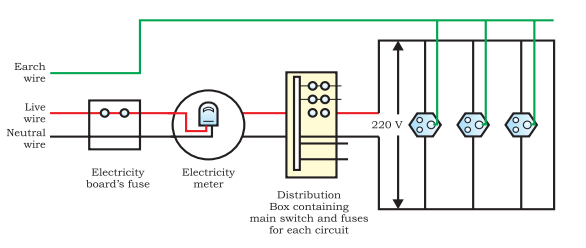link to this page by copying the following text